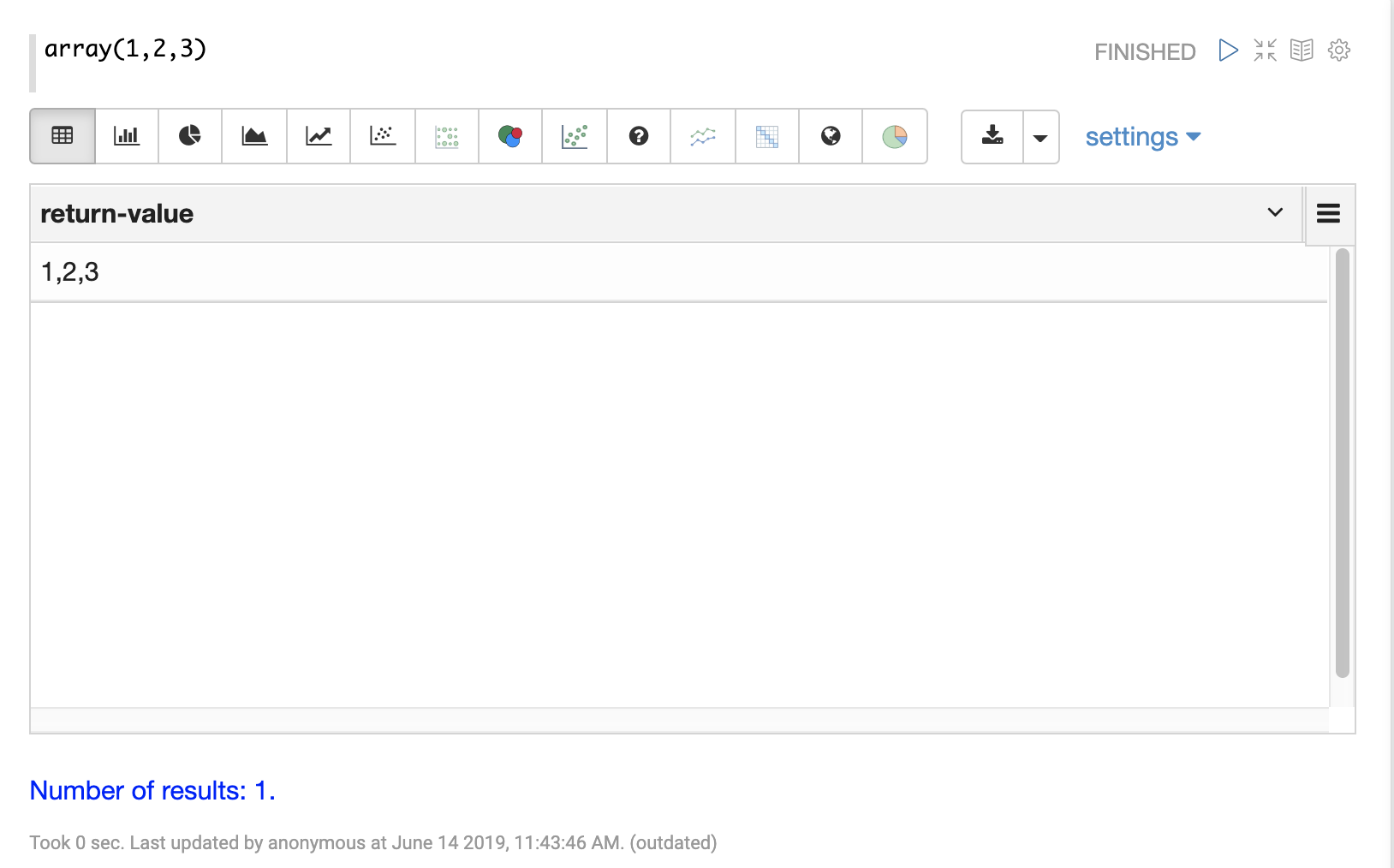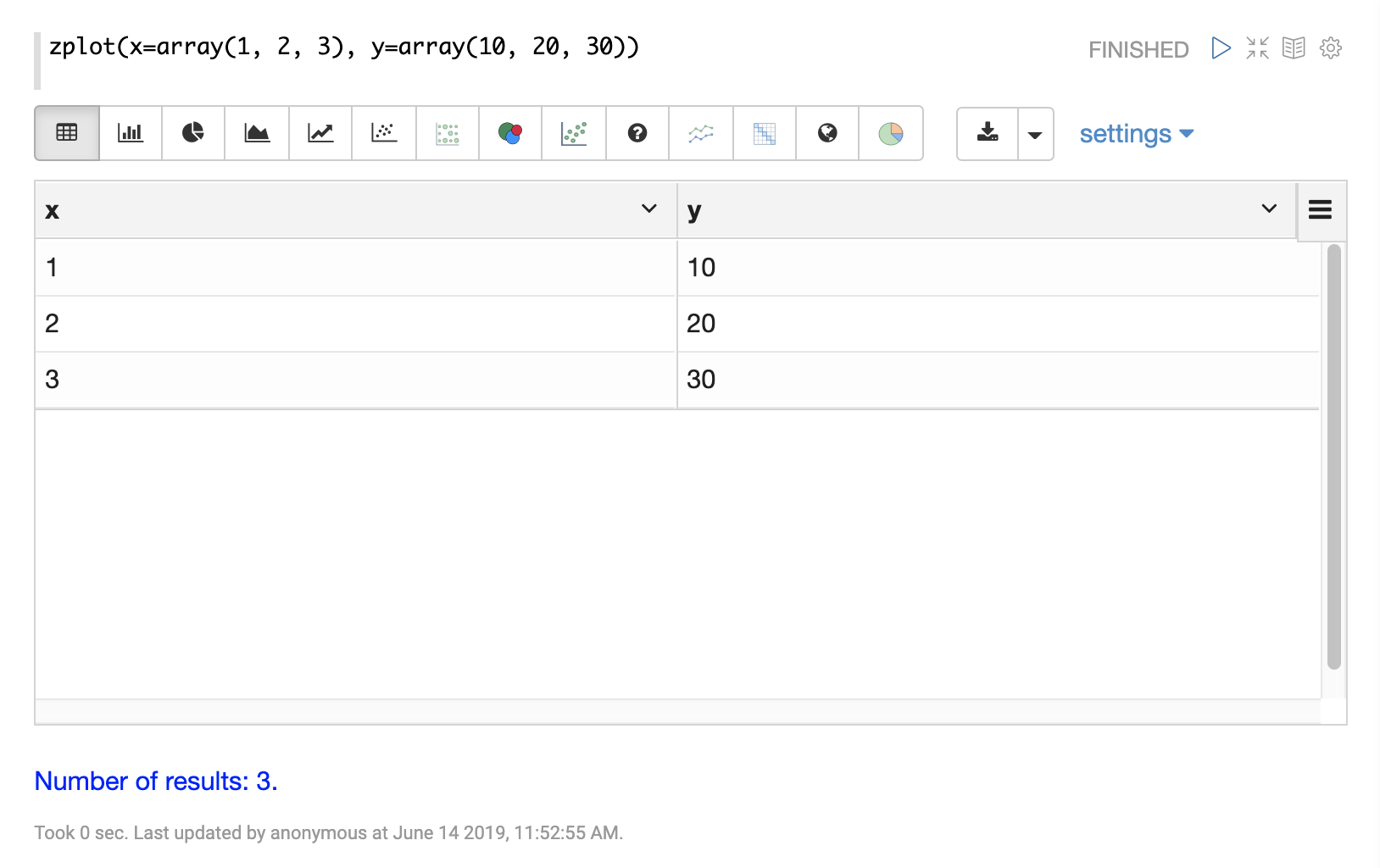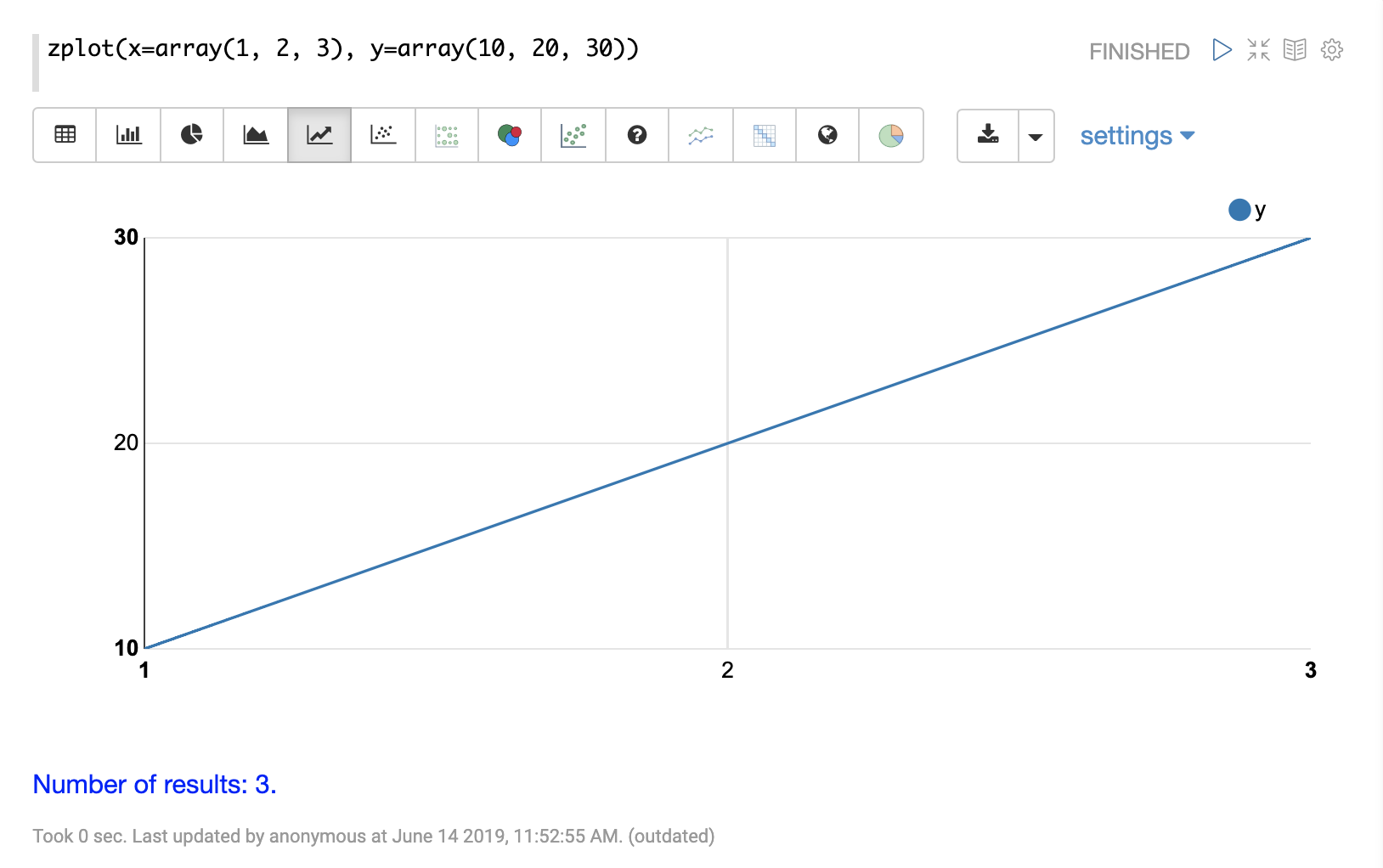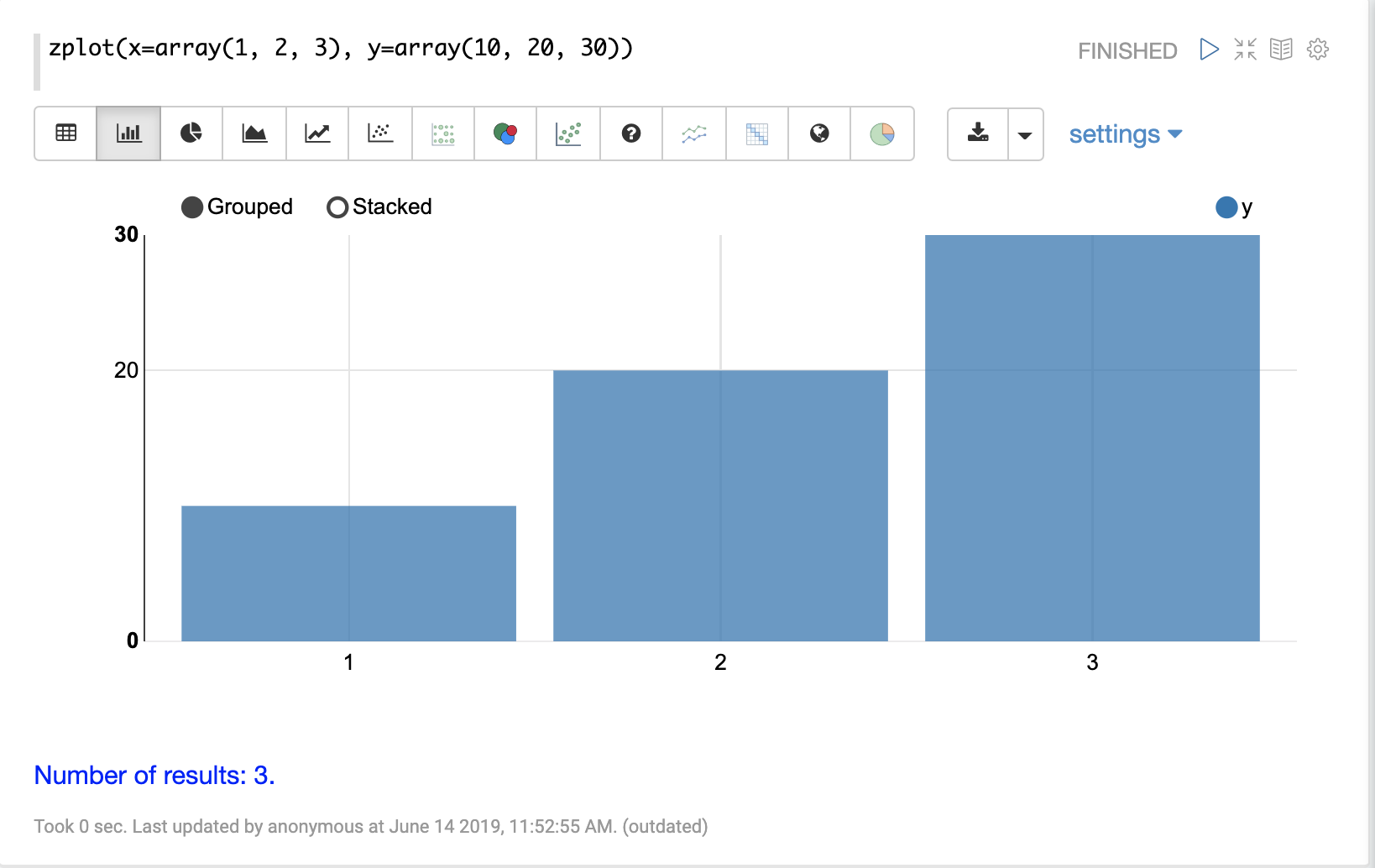# Vector Math

This section covers vector math and vector manipulation functions.

## Arrays

Arrays can be created with the `array` function.

For example, the expression below creates a numeric array with three elements:

``array(1, 2, 3)``

When this expression is sent to the `/stream` handler it responds with a JSON array:

``````{
"result-set": {
"docs": [
{
"return-value": [
1,
2,
3
]
},
{
"EOF": true,
"RESPONSE_TIME": 0
}
]
}
}``````

## Visualization

The `zplot` function can be used to visualize vectors using Zeppelin-Solr.

Let’s first see what happens when we visualize the array function as a table.It appears as one row with a comma-delimited list of values. You’ll find that you can’t visualize this output using any of the plotting tools.

To plot the array you need the `zplot` function. Let’s first look at how `zplot` output looks like in JSON format.

``zplot(x=array(1, 2, 3))``

When this expression is sent to the `/stream` handler it responds with a JSON array:

``````{
"result-set": {
"docs": [
{
"x": 1
},
{
"x": 2
},
{
"x": 3
},
{
"EOF": true,
"RESPONSE_TIME": 0
}
]
}
}``````

`zplot` has turned the array into three tuples with the field `x`.

``zplot(x=array(1, 2, 3), y=array(10, 20, 30))``

When this expression is sent to the `/stream` handler it responds with a JSON array:

``````{
"result-set": {
"docs": [
{
"x": 1,
"y": 10
},
{
"x": 2,
"y": 20
},
{
"x": 3,
"y": 30
},
{
"EOF": true,
"RESPONSE_TIME": 0
}
]
}
}``````

Now we have three tuples with `x` and `y` fields.

Let’s see how Zeppelin-Solr handles this output in table format:Now that we have `x` and `y` columns defined we can simply switch to one of the line charts and plugin the fields to plot using the chart settings:Each chart has settings which can be explored by clicking on settings.

You can switch between chart types for different types of visualizations. Below is an example of a bar chart:## Array Operations

Arrays can be passed as parameters to functions that operate on arrays.

For example, an array can be reversed with the `rev` function:

``rev(array(1, 2, 3))``

When this expression is sent to the `/stream` handler it responds with:

``````{
"result-set": {
"docs": [
{
"return-value": [
3,
2,
1
]
},
{
"EOF": true,
"RESPONSE_TIME": 0
}
]
}
}``````

Another example is the `length` function, which returns the length of an array:

``length(array(1, 2, 3))``

When this expression is sent to the `/stream` handler it responds with:

``````{
"result-set": {
"docs": [
{
"return-value": 3
},
{
"EOF": true,
"RESPONSE_TIME": 0
}
]
}
}``````

A slice of an array can be taken with the `copyOfRange` function, which copies elements of an array from a start and end range.

``copyOfRange(array(1,2,3,4,5,6), 1, 4)``

When this expression is sent to the `/stream` handler it responds with:

``````{
"result-set": {
"docs": [
{
"return-value": [
2,
3,
4
]
},
{
"EOF": true,
"RESPONSE_TIME": 0
}
]
}
}``````

Elements of an array can be trimmed using the `ltrim` (left trim) and `rtrim` (right trim) functions. The `ltrim` and `rtrim` functions remove a specific number of elements from the left or right of an array.

The example below shows the `lrtim` function trimming the first 2 elements of an array:

``ltrim(array(0,1,2,3,4,5,6), 2)``

When this expression is sent to the `/stream` handler it responds with:

``````{
"result-set": {
"docs": [
{
"return-value": [
2,
3,
4,
5,
6,
]
},
{
"EOF": true,
"RESPONSE_TIME": 1
}
]
}
}``````

## Getting Values By Index

Values from a vector can be retrieved by index with the `valueAt` function.

``valueAt(array(0,1,2,3,4,5,6), 2)``

When this expression is sent to the `/stream` handler it responds with:

``````{
"result-set": {
"docs": [
{
"return-value": 2
},
{
"EOF": true,
"RESPONSE_TIME": 0
}
]
}
}``````

## Sequences

The `sequence` function can be used to generate a sequence of numbers as an array. The example below returns a sequence of 10 numbers, starting from 0, with a stride of 2.

``sequence(10, 0, 2)``

When this expression is sent to the `/stream` handler it responds with:

``````{
"result-set": {
"docs": [
{
"return-value": [
0,
2,
4,
6,
8,
10,
12,
14,
16,
18
]
},
{
"EOF": true,
"RESPONSE_TIME": 7
}
]
}
}``````

The `natural` function can be used to create a sequence of natural numbers starting from zero. Natural numbers are positive integers.

The example below creates a sequence starting at zero with all natural numbers up to, but not including 10.

``natural(10)``

When this expression is sent to the `/stream` handler it responds with:

``````{
"result-set": {
"docs": [
{
"return-value": [
0,
1,
2,
3,
4,
5,
6,
7,
8,
9
]
},
{
"EOF": true,
"RESPONSE_TIME": 0
}
]
}
}``````

## Vector Sorting

An array can be sorted in natural ascending order with the `asc` function.

The example below shows the `asc` function sorting an array:

``asc(array(10,1,2,3,4,5,6))``

When this expression is sent to the `/stream` handler it responds with:

``````{
"result-set": {
"docs": [
{
"return-value": [
1,
2,
3,
4,
5,
6,
10
]
},
{
"EOF": true,
"RESPONSE_TIME": 1
}
]
}
}``````

## Vector Summarizations and Norms

There are a set of functions that perform summarizations and return norms of arrays. These functions operate over an array and return a single value. The following vector summarizations and norm functions are available: `mult`, `add`, `sumSq`, `mean`, `l1norm`, `l2norm`, `linfnorm`.

The example below shows the `mult` function, which multiples all the values of an array.

``mult(array(2,4,8))``

When this expression is sent to the `/stream` handler it responds with:

``````{
"result-set": {
"docs": [
{
"return-value": 64
},
{
"EOF": true,
"RESPONSE_TIME": 0
}
]
}
}``````

The vector norm functions provide different formulas for calculating vector magnitude.

The example below calculates the `l2norm` of an array.

``l2norm(array(2,4,8))``

When this expression is sent to the `/stream` handler it responds with:

``````{
"result-set": {
"docs": [
{
"return-value": 9.16515138991168
},
{
"EOF": true,
"RESPONSE_TIME": 0
}
]
}
}``````

## Scalar Vector Math

Scalar vector math functions add, subtract, multiply, or divide a scalar value with every value in a vector. The following functions perform these operations: `scalarAdd`, `scalarSubtract`, `scalarMultiply`, and `scalarDivide`.

Below is an example of the `scalarMultiply` function, which multiplies the scalar value `3` with every value of an array.

``scalarMultiply(3, array(1,2,3))``

When this expression is sent to the `/stream` handler it responds with:

``````{
"result-set": {
"docs": [
{
"return-value": [
3,
6,
9
]
},
{
"EOF": true,
"RESPONSE_TIME": 0
}
]
}
}``````

## Element-By-Element Vector Math

Two vectors can be added, subtracted, multiplied and divided using element-by-element vector math functions. The available element-by-element vector math functions are: `ebeAdd`, `ebeSubtract`, `ebeMultiply`, `ebeDivide`.

The expression below performs the element-by-element subtraction of two arrays.

``ebeSubtract(array(10, 15, 20), array(1,2,3))``

When this expression is sent to the `/stream` handler it responds with:

``````{
"result-set": {
"docs": [
{
"return-value": [
9,
13,
17
]
},
{
"EOF": true,
"RESPONSE_TIME": 5
}
]
}
}``````

## Dot Product and Cosine Similarity

The `dotProduct` and `cosineSimilarity` functions are often used as similarity measures between two sparse vectors. The `dotProduct` is a measure of both angle and magnitude while `cosineSimilarity` is a measure only of angle.

Below is an example of the `dotProduct` function:

``dotProduct(array(2,3,0,0,0,1), array(2,0,1,0,0,3))``

When this expression is sent to the `/stream` handler it responds with:

``````{
"result-set": {
"docs": [
{
"return-value": 7
},
{
"EOF": true,
"RESPONSE_TIME": 15
}
]
}
}``````

Below is an example of the `cosineSimilarity` function:

``cosineSimilarity(array(2,3,0,0,0,1), array(2,0,1,0,0,3))``

When this expression is sent to the `/stream` handler it responds with:

``````{
"result-set": {
"docs": [
{
"return-value": 0.5
},
{
"EOF": true,
"RESPONSE_TIME": 7
}
]
}
}``````